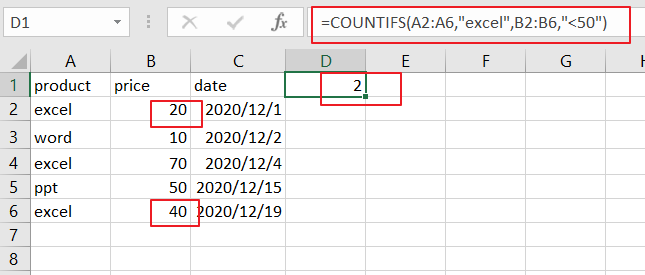# How to Count the Cells that Match Two Criteria in Excel

This post will guide you how to Count Cells that match two or more criteria in the range of dates using a formula in Excel 2013/2016 or Excel office 365.How do I count on two or multiple criteria or you want to count something in a range of cells only if two or three conditions match in Excel. And you can use the COUNTIFS function to count the number of cells in a range that match a set of multiple criteria in Excel.

The COUNTIFS function extends the COUNTIF function which only allow one criteria. And the COUNTIFS function is similar with the SUMIFS function, which can be used to find the sum of all cells that match two or more criteria.

The Syntax of COUNTIFS function is as below:

=COUNTIFS(range,criteria1,range2,criteria2,…)

Note: range is the first range of cells. Criteria1 is the criteria that be matched against range.

Range2 is the second range of cells that should be matched against criteria2.

And if all of criteria is matched in those ranges then a cell is counted.

## Count the Cells that Match Two Criteria

Assuming that you want to count the number of cells that contain “excel” and price is less than 50. And you have to count on multiple criteria based on COUNTIFS function like this:

=COUNTIFS(A2:A6,”excel”,B2:B6,”<50″)

###You can see that two different ranges should be used in the above formula.

And there is an alternative way of COUNTIFS to count that match two criteria in Excel, you can also use the SUMPRODUCT function to count cells that match multiple criteria. And the equivalent formula like this:

=SUMPRODUCT((A2:A6=”excel”)*(B2:B6<50))

### Related Functions

• Excel SUMPRODUCT function
The Excel SUMPRODUCT function multiplies corresponding components in the given one or more arrays or ranges, and returns the sum of those products. The syntax of the SUMPRODUCT function is as below:= SUMPRODUCT (array1,[array2],…)…
• Excel COUNTIF function
The Excel COUNTIF function will count the number of cells in a range that meet a given criteria. This function can be used to count the different kinds of cells with number, date, text values, blank, non-blanks, or containing specific characters.etc.= COUNTIF (range, criteria)…
• Excel COUNTIFS function
The Excel COUNTIFS function returns the count of cells in a range that meet one or more criteria. The syntax of the COUNTIFS function is as below:= COUNTIFS(criteria_range1, criteria1, [criteria_range2, criteria2]…)…
• Excel SUMIFS Function
The Excel SUMIFS function sum the numbers in the range of cells that meet a single or multiple criteria that you specify. The syntax of the SUMIFS function is as below:=SUMIFS (sum_range, criteria_range1, criteria1, [criteria_range2, criteria2], …)…